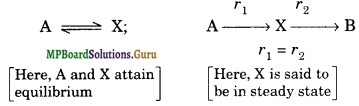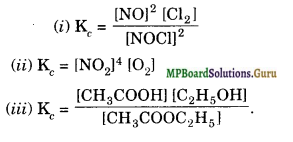# MP Board Class 11th Chemistry Solutions Chapter 7 Equilibrium

In this article, we will share MP Board Class 11th Chemistry Solutions Chapter 7 Equilibrium Pdf, These solutions are solved by subject experts from the latest edition books.

## MP Board Class 11th Chemistry Solutions Chapter 7 Equilibrium

### MP Board Class 11 Chemistry Equilibrium Textbook Questions and Answers

Question 1.
A liquid is in equilibrium with its vapour in a sealed container at a fixed temperature. The volume of the container is suddenly increased.
(a) What is the initial effect of the change on vapour pressure?
(b) How do rates of evaporation and condensation change initially?
(c) What happens when equilibrium is restored finally and what will be the final vapour pressure?
Solution.
(a) Vapour pressure decreases due to increase in volume.
(b) Rate of evaporation remains same and rate of condensation decreases.
(c) Finally the same vapour pressure is restored and the rate of evaporation becomes equal to the rate of condensation.

Question 2.
What is Kc for the following equilibrium when the equilibrium concentration of each substance is : [SO2] = 0.60 M, [O2] = 0.82 M and [SO3] = 1.90 M?
2SO2 (g) + O2 (g) ⇌ 2SO3 (g)
Solution:
Kc = $$\frac{\left[\mathrm{SO}_{3}\right]^{2}}{\left[\mathrm{SO}_{2}\right]^{2}\left[\mathrm{O}_{2}\right]}$$
= $$\frac{(1.90)^{2}}{(0.60)^{2}(0.82)}$$
= 12.23 mol L1.

Question 3.
At a certain temperature and total pressure of 105 Pa, iodine vapour contains 40% by volume of I atoms
I2(g) ⇌ 2l(g)
Calculate Kp for the equilibrium.
Solution.
The number of moles of I2 molecules and I atoms are proportional to their volumes.
∴ Ratio of moles of I2 and I is 60 : 40 or 3:2 3
Mole fraction of I2 = $$\frac{3}{5}$$
Mole fraction of I = $$\frac{2}{5}$$
Partial pressure of I2, PI2 = Total pressure x mole fraction of I2
= 105 × $$\frac{3}{5}$$ = 6 × 104 Pa
Partial pressure of I atoms, p1 = Total pressure x mole fraction of I
= 105 × $$\frac{2}{5}$$ = 4 × 104 Pa
Expression for Kp for the reaction, I2(g) ⇌ 2I (g) may be written as
Kp = $$\frac{\left(p_{\mathrm{I}}\right)^{2}}{p_{\mathrm{I}_{2}}}$$
= $$\frac{\left(4 \times 10^{4}\right)^{2}}{6 \times 10^{4}}$$
= 2.67 × 104.

Question 4.
Write the expression for the equilihrium constant, Kc for each of the following reactions :
(i) 2NOCl(g) ⇌2NO(g) + Cl2(g)
(ii) 2CU(NO3)2(S) ⇌ 2CuO(s) + 4NO2(g) + O2(g)
(iii) CH3COOC2H5(aq) + H2O(l) ⇌ CH3COOH(aq) + C2H5OH(aq)
(iv) Fe3+(aq) + 3OH(aq)
(v) I2(s) + 5F2(g) ⇌ 2IF5(I).
Solution: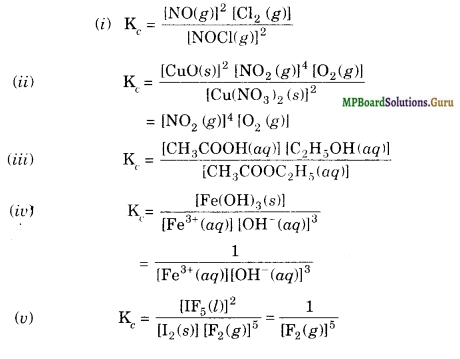Question 5.
Find out value of Kc for each of the following equilibria from the value of K :
(а) 2NOCl(g) ⇌ 2NO(g) + Cl2(g), Kp= 1.8 × 10-2 at 500 K P
(b) CaC03(s) ⇌ CaO(s) + CO2(g), Kp = 167 at 1073 K.
Solution.
(a) 2N0Cl (g) ⇌ 2N0(g) + Cl2(g)
Kp = 1.8 × 10-2
Δn(g)=3-2=1
Kp= Kc(RT)Δn = Kc(RT)
= $$\frac{1.8 \times 10^{-2}}{0.0831 \times 500}$$
= 4.33 x 10-4

(b) CaCO3(s) ⇌ CaO (s) + CO2 (g)
Kp = 167
Δng = 1
Kp = Kc (RT)Δn
= Kc (RT)
Kc = $$\frac{\mathrm{K}_{p}}{\mathrm{RT}}$$
= $$\frac{167}{0.0831 \times 1073}$$
= 1.88.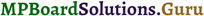Question 6.
For the following equilibrium, Kc = 6.3 × 1014 at 1000 K.
NO(g) + O3(g) ⇌ NO2(g) + O2(g)
Both the forward and reverse reactions in the equilibrium are elementary bimolecular reactions. What is Kc for the reverse reaction?
Solution.
For the reverse reaction
Kc = $$\frac{1}{\mathrm{~K}_{\mathrm{c}}}$$
= $$\frac{1}{6.3 \times 10^{14}}$$
= 1.59 × 1015.

Question 7.
Explain why pure liquids and solids are ignored while writing the equilibrium constant expression.
Solution.
[Pure liquid ] or [Pure solid] = $$\frac{\text { No. of moles }}{\text { Volume }}$$ = $$\frac{\text { Mass }}{\text { Mol. mass } \times \text { Volume }}$$
= $$\frac{\text { Mass }}{\text { Volume }} \times \frac{1}{\text { Mol. mass }}$$
= $$\frac{\text { Density }}{\text { Mol. mass }}$$
As density of a pure liquid or pure solid is constant at constant temperature and molecular mass is also constant, therefore, their molar concentrations are constant and are independent of the amount of the substance present. Hence, pure liquids and solids are ignored while writing an equilibrium constant expression.

Question 8.
Reaction between N2 and O2 takes place as follows :
2N2(g) + O2(g) ⇌ 2N2O(g) .
If a mixture of 0.482 mol N2 and 0.933 mol of O2 is placed in a 10 L reaction vessel and allowed to form N2O at a temperature for which Kc = 2.0 × 10-37, determine the composition of equilibrium mixture.
Solution.
2N2(g) + O2(g) ⇌ 2N2O(g) .
Initial moles : 0.482 0.933
Moles at equilibrium: (0.482 – x) (0.933 — x/2) x
Since the value of K is very small (2.0 × 10-37) it indicates that the equilibrium is very much in favour of reactants and thus the value of x is very very small. Therefore, the approximate concentrations at equilibrium may be written as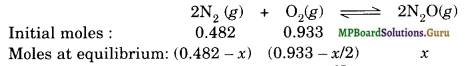x = 6.6 × 10-20
∴ [N2O] = 0.1 × 6.6 × 10-20 = 6.6 × 10-21 mol L-1
[N2] = 0.0482 mol L-1
[O2] = 0.0933 mol L-1.

Question 9.
Nitric oxide reacts with Br2 and gives nitrosyl bromide as per reaction given below:
2NO(g) + Br2(g) ⇌ 2NOBr(g) When 0.087 mol of NO and 0.0437 mol of Br2 are mixed in a closed container at constant temperature, 0.0518 mol of NOBr is obtained at equilibrium. Calculate equilibrium amount of NO and Br2.
Solution.
2NO(g) + Br2(g) ⇌ 2NOBr(g)
2 mol of NO react with 1 mol of Br2 to form 2 mol NOBr. 0.0518 mol of NOBr would be formed by reaction of 0.0518 mol of NO and 0.0518/2 mol of Br2.
Amount of NO left unreacted
= (0.087 – 0.0518) mol = 0.0352 mol
Amount of Br2 left unreacted
= (0.0437 – 0.0518/2) mol = 0.0178 mol.

Question 10.
At 450 K, Kp = 2.0 × 1010bar for the given reaction at equilibrium
2SO2(g) + O2(g)⇌2SO3(g)
What is Kc at this temperature ?
Solution.
For the given reaction,
Δng = (np-nr) = 2 – 3 = – 1
Kp = Kc (RT)Δn
or Kc = Kp (RT)-Δn
= Kp (RT)
= (2.0 × 1010 bar-1) (0.0831 L bar K-1 mol-1) (450 K)
= 7.48 × 1011 L mol-1.

Question 11.
A sample of HI(g) is placed in flask at a pressure of 0.2 atm. At equilibrium, the partial pressure of HI(g) is 0.04 atm. What is Kp for the given equilibrium?
2HI(g) ⇌ H2(g) + I2(g)
Solution.
2HI (g) ⇌ H2(g) + I2(g)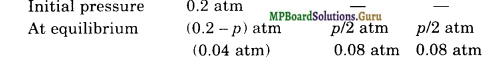Kp = $$\frac{\mathrm{p}_{\mathrm{H}_{2}} \times \mathrm{p}_{1_{2}}}{\left(\mathrm{p}_{\mathrm{H} \mathrm{I}}\right)^{2}}$$
= $$\frac{0.08 \times 0.08}{(0.04)^{2}}$$
= 4.

Question 12.
A mixture of 1.57 mol of N2 , 1.92 mol of H2 and 8.13 mol of NH3 is introduced into a 20 L reaction vessel at 500 K. At this temperature, the equilibrium constant, Kc for the reaction
N2(g) + 3H2(g)⇌2 NH3(g) is 1.7 × 102.
Is the reaction mixture at equilibrium? If not, what is the direction of the net reaction?
Solution:
N2(g) + 3H2(g)⇌2 NH3(g)
Qc = $$\frac{\left[\mathrm{NH}_{3}\right]^{2}}{\left[\mathrm{~N}_{2}\right]\left[\mathrm{H}_{2}\right]^{3}}$$
= $$\frac{\left(8.13 / 20 \mathrm{~mol} \mathrm{~L}^{-1}\right)^{2}}{\left(1.57 / 20 \mathrm{~mol} \mathrm{~L}^{-1}\right)\left(1.92 / 20 \mathrm{~mol} \mathrm{~L}^{-1}\right)^{3}}$$
= 2.38 × 103
Since Qc ≠ Kc, the reaction mixture is not at equilibrium.
Since Qc > Kc, the net reaction will be in the backward direction.Question 13.
The equilibrium constant expression for a gas reaction is
Kc = $$\frac{\left[\mathrm{NH}_{3}\right]^{4}\left[\mathrm{O}_{2}\right]^{5}}{[\mathrm{NO}]^{4}\left[\mathrm{H}_{2} \mathrm{O}\right]^{6}}$$
Write the balanced chemical equation corresponding to this expression.
Solution.
4NO(g) + 6H2O(g) → 4NH3(g) + 5O2(g).

Question 14.
One mole of H2O and one mole of CO are taken in 10 L vessel and heated to 725 K. At equilibrium 40% of water (by mass) reacts with CO according to the equation,
H2O(g) + CO(g) ⇌ H2(g) + CO2(g)
Calculate the equilibrium constant for the reaction.
Solution.
At equilibrium,
[H2O] = $$\frac{1-0.40}{10}$$ mol L-1 = 0.06 mol L-1
[COl = 0.06 mol L-1,
[H2] = $$\frac{0.40}{10}$$ mol L-1 = 0.04 mol L-1,
[CO2] = 0.04 mol L-1
K = $$\frac{\left[\mathrm{H}_{2}\right]\left[\mathrm{CO}_{2}\right]}{\left[\mathrm{H}_{2} \mathrm{O}\right][\mathrm{CO}]}$$
= $$\frac{0.04 \times 0.04}{0.06 \times 0.06}$$
= 0.44.

Question 15.
At 700 K, equilibrium constant for the reaction:
H2(g) + I2(g) ⇌ 2HI(g) is 54.8. If 0.5 mol L-1 of HI(g) is present at equilibrium at 700 K, what are the concentrations of H2(g) and I2(g) assuming that we initially started with HI(g) and allowed it to reach equilibrium at 700 K?
Solution.
H2(g) + I2(g) ⇌ 2HI(g)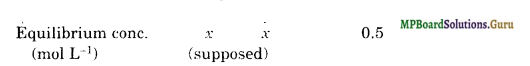K = $$\frac{[\mathrm{HI}]^{2}}{\left[\mathrm{H}_{2}\right]\left[\mathrm{I}_{2}\right]}$$
= $$\frac{(0.5)^{2}}{(x)(x)}$$
= 54.8
x = 0.068
[H2] = 0.068 mol L-1
[ I2] = 0.068 mol L-1.

Question 16.
What is the equilibrium concentration of each substance in the equilibrium when the initial concentration of iCI was 0.78 M?
2ICI(g) ⇌ I2(g) + Cl2(g); Kc = 0.14
Solution.
Suppose at equilibrium,
[I2] = [Cl2] = x mol L-1
2ICI(g) ⇌ I2(g) + Cl2(g)
img
Kc = $$\frac{\left[\mathrm{I}_{2}\right]\left[\mathrm{Cl}_{2}\right]}{[\mathrm{ICl}]^{2}}$$
∴ 0.14 = $$\frac{x \times x}{(0.78-2 x)^{2}}$$
or $$\frac{x}{0.78-2 x}$$ = $$\sqrt{0.14}$$ = 0.37
or x = 0.29 – 0.74 x
or 1.74 x = 0.29 or x = 0.17
Hence, at equilibrium
[I2] = [Cl2] = 0.17 M [ICI]
= 0.78 – 2 × 0.17 M
= 0.44 M.

Question 17.
Kp = 0.04 atm at 899 K for the equilibrium shown below. What is the equilibrium concentration of C2H6 when it is placed in a flask at 4.0 atm pressure and allowed to come to equilibrium?
C2H6(g) ⇌ C2H4(g) + H2(g)
Solution.
C2H6(g) ⇌ C2H4(g) + H2(g)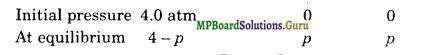Kp = $$\frac{P_{\mathrm{C}_{2} \mathrm{H}_{\mathrm{L}}} \times P_{\mathrm{H}_{2}}}{P_{\mathrm{C}_{2} \mathrm{H}_{6}}}$$
∴ 0.04 = $$\frac{p \times p}{4-p}$$ = p2 = 0.16 – 0.04 p
or p2+ 0.04 p -0.16 = 0
p = 0.40
∴ [C2H6]eq = (4 -0.40) atm = 3.60 atm.

Question 18.
Ethyl acetate is formed by the reaction between ethanol and acetic acid and the equilibrium is represented as:
CH3COOH(l) + C2H5OH(l) ⇌ CH3COOC2H5(I) + H2O(l)
(i) Write the concentration ratio (reaction quotient), for this reaction (note: water is not in excess and is not a solvent in this reaction).
(ii) At 293 K. if one starts with 1.00 mol of acetic acid and 0.18 mol of ethanol, there is 0.171 mol of ethyl acetate in the final equilibrium mixture. Calculate the equilibrium constant.
(iii) Starting with 0.5 mol of ethanol and 1.0 mol of acetic acid and maintaining it at 29 K, G.214 mol of ethyl acetate is found after some time. Has equilibrium been reached?
Solution.
(i) Qc = $$\frac{\left[\mathrm{CH}_{3} \mathrm{COOC}_{2} \mathrm{H}_{5}\right]\left[\mathrm{H}_{2} \mathrm{O}\right]}{\left[\mathrm{C}_{3} \mathrm{COOH}\right]\left[\mathrm{C}_{2} \mathrm{H}_{5} \mathrm{OH}\right]}$$

(ii) CH3COOH(l) + C2H5OH(l) ⇌ CH3COOC2H5(I) + H2O(l)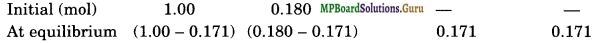Kc = $$\frac{(0.171 / \mathrm{V})(0.171 / \mathrm{V})}{(0.829 / \mathrm{V})(0.009 / \mathrm{V})}$$
= $$\frac{(0.171)(0.171)}{(0.829)(0.009)}$$
= 3.92

(iii) CH3COOH(l) + C2H5OH(l) ⇌ CH3COOC2H5(I) + H2O(l)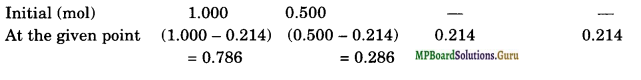Qc = $$\frac{(0.214)(0.214)}{(0.786)(0.286)}$$
= 0.204
Since Qc is less than Kc, therefore, the equilibrium is not reached.

Question 19.
A sample of pure PCl5 was introduced into an evacuated vessel at 473 K. After equilibrium was attained, concentration of PCl5 was found to be 0.5 × 10-1 mol L-1. If value of is 8.3 × 10-3 what are the concentrations of PCl3 and Cl2 at equilibrium?
Solution.
PCl5(g) ⇌ PCl3(g) + Cl2(g)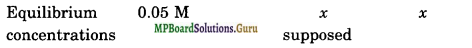Kc = $$\frac{\left[\mathrm{PCl}_{3}\right]\left[\mathrm{Cl}_{2}\right]}{\left[\mathrm{PCl}_{5}\right]}$$
8.3 × 10-3= $$\frac{(x)(x)}{(0.05)}$$
x2 = 8.3 × 10-3 × 0.05
x = 2 × 10-2
∴ [pcl5] = 2 × 10-2 M
[cl2] = 2 × 10-2 M.

Question 20.
One of the reactions that takes place in producing steel from iron ore is the reduction of iron (II) oxide by carbon monoxide to give iron metal and CO2.
FeO(s) + CO(g) ⇌Fe(s) + CO2 (g);
Kp= 0.265 atm at 1050 K.
What are the equilibrium partial pressures of CO and CO2 at 1050 K if the initial pressures are: Pco= 1.4 atm and pco2 = 0.80 atm?
Solution.
FeO(s) + CO(g) ⇌Fe(s) + CO2 (g)
Initial pressures – 1.4 atm – 0.80 atm.
Qp = $$\frac{p_{\mathrm{CO}_{2}}}{p_{\mathrm{CO}}}$$
= $$\frac{0.80}{1.4}$$
= 0.571

Since Qp > Kp, the net reaction will proceed in the backward direction. Therefore, pressure of CO2 will decrease and that of CO will increase to attain equilibrium. Hence, if p is the decrease in pressure of CO2, then increase in pressure of CO = p.
∴ At equilibrium,
Pco2 = (0.80 -p) atm,pco = (1.4 +p) atm
Kp = $$\frac{p_{\mathrm{CO}_{2}}}{p_{\mathrm{CO}}}$$
∴ 0.265 = $$\frac{0.80-p}{1.4+p}$$ or 0.265 (1.4 +p) = 0.80 – p
or 0.371 + 0.265 p = 0.80 – p
or 1.265 p = 0.429 or p = 0.339 atm
∴ At equilibrium:
pco = 1.4 + 0.339 atm = 1.739 atm
Pco2 = 0.80 – 0.339 atm = 0.461 atm.

Question 21.
Equilibrium constant, Kc for the reaction
N2(g) + 3H2g ⇌ 2NH3(g) at 500 K is 0.061
At a particular time, the analysis shows that composition of the reaction mixture is 3.0 mol L-1 N2, 2.0 mol L-1 H2 and 0.5 mol L-1 NH3. Is the reaction at equilibrium? If not in which direction would the reaction tend to proceed to reach equilibrium?
Solution.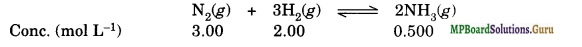Since Qc is not equal to Kc, the reaction is not at equilibrium.
Since Qc is less than Kc, the reaction would tend to proceed in forward direction to attain equilibrium.

Question 22.
Bromine monochloride, BrCl decompose into bromine and chlorine and reaches the equilibrium:
2BrCl(g) ⇌ Br2(g) + Cl2(g)
for which Kc = 32 at 500 K. If initially pure BrCl is present at a concentration of 3.3 x 10-3 mol L-1, what is its molar concentration in the mixture at equilibrium?
Solution.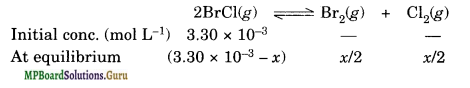Taking square root of both sides
5.66 = $$\frac{x / 2}{\left(3.30 \times 10^{-3}-x\right)}$$
11.32(3.30 x 10-3 – x) = x
x = 3.0 x 10-3
∴ At equilibrium
[BrCl] = (3.30 x 10-3 – 3.0 x 10-3 )
= 0.30 x 10-3
= 3.0 x 10-4 mol L-1.

Question 23.
At 1127 K and 1 atm pressure, a gaseous mixture of CO and CO2 in equilibrium with solid carbon has 90.55% CO by mass
C(s) + CO2(g) ⇌ 2CO(g)
Calculate Kc for this reaction at the above temperature.
Solution.
If total mass of the mixture of CO and CO2 is 100 g, then
Mass of CO = 90.55 g and mass of CO2 = 100 – 90.55 = 9.45 g
∴ Number of moles of CO = 90.55/28 = 3.23
Number of moles of CO2 = $$\frac{9.45}{44}$$
∴ PCo = $$\frac{3.23}{3.23+0.22}$$ x 1 atm = 0.94 atm
PCo2 = $$\frac{0.22}{3.23+0.22}$$ x 1atm = 0.06 atm

Kp = $$\frac{p^{2} \mathrm{cO}}{p_{\mathrm{CO}_{2}}}=\frac{(0.94)^{2}}{0.06}$$ = 14.27
Δng = 2 – 1 = 1
∴ Kp = Kc(RT)
or Kc = $$\frac{\mathrm{K}_{p}}{\mathrm{RT}}$$
= 
= 0.154.

Question 24.
Calculate (a) ΔG° and (b) the equilibrium constant for the formation of NO2 from NO and O2 at 298 K
NO(g) + $$\frac { 1 }{ 2 }$$O2(g) ⇌ NO2(g)
where ∆fG° (NO2) = 52.0 kJ/mol, ∆fG° (NO) = 87.0 kJ/mol, ∆fG°(O2) = 0 kJ/mol.
Solution:
(a) ∆rG° = Σ∆fG°(Products) – Σ∆fG°(Reactants)
fG° (NO2) – [∆fG°(NO) + $$\frac { 1 }{ 2 }$$∆fG°(O2)]
= 52.0 – ( 87.0 + $$\frac { 1 }{ 2 }$$ x 0 ) = – 35.0 kJ mol-1

(b) – ΔG° = 2.303 RT log K.
∴ – (- 35000) = 2.303 x 8.314 x 298 x log K
or . log K= 6.1341
K = Antilog 6.1341
K = 1.361 x 106.

Question 25.
Does the number of moles of reaction products increase, decrease or remain same when each of the following equilibria is subjected to a decrease in pressure by increasing the volume?
(a) PCl5(g) ⇌PCl3(g) + Cl2(g)
(b) CaO(s) + CO2(g) ⇌ CaCO3(s)
(c) 3Fe(s) + 4H2O(g) ⇌ Fe3O4(s) + 4H2(g).
Solution.
Applying Le-Chatelier’s principle, on decreasing the pressure, moles of reaction products will
(a) increase
(b) decrease
(c) remain same.Question 26.
Which of the following reactions will get affected by increasing the pressure?
Also mention whether change will cause the reaction to go into forward or backward direction.
(i) COCl2(g) ⇌ CO(g) + Cl2(g)
(ii) CH4(g) + 2S2(g) ⇌ CS2(g) + 2H2S(g)
(iii) CO2(g) + C(s) ⇌ 2CO(g)
(iv) 2H2(g) + CO(g)⇌ CH3OH(g)
(v) CaCO3(s) ⇌ CaO(s) + CO2(g)
(vi) 4NH3 (g) + 5O2(g) ⇌ 4NO(g) + 6H2O(g).
Solution.
The reactions in which the number of moles of gaseous reactants is not equal to the number of moles of gaseous products (n ) will be affected by increasing the pressure. Thus, the reactions (i), (iii), (iv), (y) anð(vi) will be affected. According to Le-Chatelier’s principle, on increasing the pressure, the equilibrium will shift in the direction where number of moles of gaseous species is less.
(i) np = 2, nr. = 1, the reaction will go in the backward direction.
(ii) np = 3, nr. = 3, since np = nr, the reaction will not be affected by pressure.
(iii) np = 2, nr. = 1, the reaction will go in the backward direction.
(iv) np = 1, nr. = 3, the reaction will go in the forward direction.
(u) np = I, nr. = O, the reaction will go in the ‘backward direction.
(vi) np = 10, nr = 9, the reaction will go in the backward direction.

Question 27.
The equilibrium constant for the following reaction is 1.6 × 105 at 1024 K, H2(g) + Br2(g) ⇌ 2HBr(g)
Find the equilibrium pressure of all gases if 10.0 bar of HBr is introduced into a sealed container at 1024 K.
Solution:
H2(g) + Br2(g) ⇌ 2HBr(g)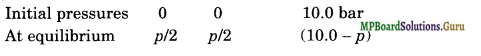Kp = $$\frac{p^{2} \mathrm{HBr}}{p_{\mathrm{H}_{2}} \times p_{\mathrm{Br}_{2}}}$$
1.6 × 105 = $$\frac{(10-p)^{2}}{(p / 2)(p / 2)}$$
Taking Square root of the both sides
4 × 102 = $$\frac{10-p}{p / 2}$$
200 p = 10 – p
p = $$\frac{10}{201}$$ bar
pH2 = p/2 = $$\frac{1}{2}\left(\frac{10}{201}\right)$$ bar = 2.5 × 10-2 bar
pBr2 = p/2 = 2.5 ×10-2 bar
pHBr = 10 – p = 10 bar.

Question 28.
Hydrogen gas is obtained from natural gas by partial oxidation with steam as per following endothermic reaction.
CH4 (g) + H2O(g) ⇌ CO(g) + 3H2(g);
(а) Write an expression for Kp for the above reaction.
(b) How will the value of Kp and composition of equilibrium mixture be affected by
(i) increasing the pressure
(ii) increasing the temperature
(iii) using a catalyst?
Solution:
(a) Kp = $$\frac{p_{\mathrm{CO} \times} p_{\mathrm{H}_{2}}^{3}}{p_{\mathrm{CH}_{4} \times} p_{\mathrm{H}_{2} \mathrm{O}}}$$
(b) (i) The value of Kpremains unchanged on increasing the pressure. When pressure is increased then according to Le-Chatelier’s principle the equilibrium shifts in the direction where there is less number of moles of gases i.e., backward direction in case of the given reaction.
(ii) In case of endothermic reactions the value of Kp increases with increases in temperature. With increases in temperature, the equilibrium shifts in the endothermic direction i.e., forward direction in case of the given reaction.
(iii) K will remain undisturbed. Equilibrium composition will remain unchanged. However, in the presence of catalyst, the equilibrium would be attained quickly.

Question 29.
Describe the effect of:
(c) removal of CO
(d) removal of CH3OH,
on the equilibrium of the reaction :
2H2(g) + CO(g) ⇌ CH3OH(g)
Solution.
(a) The equilibrium will shift in the forward direction.
(b) The equilibrium will shift in the backward direction.
(c) The equilibrium will shift in the backward direction.
(d) The equilibrium will shift in the forward direction.

Question 30.
At 473 K, equilibrium constant, Kc for decomposition of phosphorus pentachloride, PCl5 is 8.3 × 10-3. If decomposition is depicted as
PCl5(s) ⇌ PCl3(g) + Cl2(g); ΔrHθ = 124.0 kJ mol-1
(а) Write an expression for Kc for the reaction.
(b) What is the value of Kc for the reverse reaction at the same temperature?
(c) What would be the effect on Kc if (i) more PCl5 is added (ii) the pressure is increased
(iii) the temperature is increased?
Solution.
(a) Kc = $$\frac{\left[\mathrm{PCl}_{3}\right]\left[\mathrm{Cl}_{2}\right]}{\left[\mathrm{PCl}_{5}\right]}$$
= 8.3 × 10-3
(b) Kc for reverse reaction
= $$\frac{1}{K_{c} \text { for forward reaction }}$$
= $$\frac{1}{8.3 \times 10^{-3}}$$ = 120.48

(c)

(i) Kc remains unchanged = when more PCl5 is added.
(ii) When pressure is increased Kc remains unchanged.
(iii) Kc increases with increase in temperature because the reaction is endothermic.Question 31.
Dihydrogen gas used in Haber’s process is produced by reacting methane from natural gas with high-temperature steam. The first stage of two-stage reaction involves the formation of CO and H2. In second stage, CO formed in first stage is reacted with more steam in water gas shift reaction, CO(g) + H2O(g) ⇌ CO2(g) + H2(g).
If a reaction vessel at 400°C is charged with an equimolar mixture of CO and steam such that pco = pH2O Q = 4.0 bar, what will be the partial pressure of H2 at equilibrium? Kp = 10.1 at2400°C.
Solution.
Suppose the partial pressure of H2 at equilibrium = p bar
CO(g) + H2O(g) ⇌ CO2(g) + H2(g).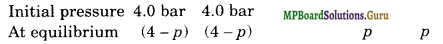Kp = $$\frac{p^{2}}{(4-p)^{2}}$$ = 10.1 (Given)
∴ $$\frac{p}{4-p}$$ = $$\sqrt{10.1}$$ = 3.18
p = 3.04 bar.

Question 32.
Predict which of the following reaction will have appreciable concentration of reactants and products :
(a) Cl2(g) ⇌ 2Cl(g), Kc = 5 × 10-39
(6) Cl2 (g) + 2NO (g) ⇌ 2 NOCl (g), Kc= 3.7 × 108
(c) Cl2 (g) + 2 NO2 (g) ⇌ 2 NO2 Cl (g), Kc= 1.8.
Solution.
For reaction (c), Kc is neither very high nor very low. Therefore, in this reaction at equilibrium reactants and products will be present in comparable amounts.

Question 33.
The value of Kc for the reaction, 3O2(g) ⇌ 2O3(g), is 2.0 × 10-50 at 25°C. If the
equilibrium concentration of O2 in air at 25°C is 1.6 × 10-2 what is the concentration of O3?
Solution.
Kc = $$\frac{\left[\mathrm{O}_{3}\right]^{2}}{\left[\mathrm{O}_{2}\right]^{3}}$$
∴ 2.0 × 10-50 = $$\frac{\left[\mathrm{O}_{3}\right]^{2}}{\left(1.6 \times 10^{-2}\right)^{3}}$$
or [O3]2 = (2.0 × 10-50) (1.6 × 10-2)3 = 8.19 × 10-56
or [O3] = 2.86 × 10-28 M.

Question 34.
The reaction, CO(g) + 3 H2(g) ⇌ CH4(g) + H2O(g), is at equilibrium at 1300 K in a 1L flask. It also contains 0.30 mol of CO, 0.10 mol of H2 and 0.02 mol of H2O and an unknown amount of CH4 in the flask. Determine the concentration of CH4 in the mixture. The equilibrium constant, Kc, for the reaction at the given temperature is 3.90.
Solution:
Kc = $$\frac{\left[\mathrm{CH}_{4}\right]\left[\mathrm{H}_{2} \mathrm{O}\right]}{[\mathrm{CO}]\left[\mathrm{H}_{2}\right]^{3}}$$
Since, the reaction is carried out in a flask of 1 L capacity, the number of moles of various species are equal to their respective molar concentrations.
∴ 3.90 = $$\frac{\left[\mathrm{CH}_{4}\right][0.02]}{(0.30)(0.10)^{3}}$$
or [CH4] = 0.585 M = 5.85 × 10-2 M.

Question 35.
What is meant by the conjugate acid-base pair? Find the conjugate acid-base for the following species:
HNO2, CN, HClO4, F, OH, CO2-3 and S2-.
Solution.
An acid-base pair which differs by a proton is called conjugate acid-base pair.
NO2, HCN, ClO4, HF, H2O, HCO3 and HS.

Question 36.
Which of the following are Lewis acids? H2O, BF3, H+, NH4+.
Solution.
BF3, H+, NH4+.

Question 37.
What will be the conjugate bases for the Bronsted acids: HF, H2SO4 and HCO3.
Solution.
A conjugate base of an acid is formed by loss of H+ by the acid.
∴ Conjugate bases of the given acids will be F, HSO4, CO32- respectively.

Question 38.
Write the conjugate acids for the following Bronsted bases:
NH2, NH3 and HCOO.
Solution.
A conjugate acid of a base is formed by gain of a proton (H+) by the base The conjugated acids of the given bases are NH3, NH4+, HCOOH respectively.

Question 39.
The species: H2O, HCO3, HSO4 and NH3 can act both as Bronsted acids and bases. For each case give the corresponding conjugate acid and base.
Solution.

 Species Conjugate acid Conjugate base H2O H3O+ OH– HCO– H2CO3 CO32- HSO4– H2SO4 SO42- NH3 NH4+ NH2–

Question 40.
Classify the following species into Lewis acids and Lewis bases and show how these act as Lewis acid/base:
(a) OH
(b) F
(c) H+
(d) BCl3
Solution.
(a) OH can donate electron pair. Hence, it is a Lewis base.
OH + H+ → H2O
(b) F can also donate electron pair. Hence, it is a Lewis base.
F + BF3 → BF4
(c) H+ can accept electron pair. Hence, it is a Lewis acid.
H+ + OH → H2O
(d) BCl3 can accept electron pair and therefore, it is a Lewis acid.
BCl3 + NH3 → HN→ BCl3.

Question 41.
The concentration of hydrogen ion in a sample of soft drink is 3.8 × 10-3 M. What is its pH?
Solution.
pH = – log [H+] = – log (3.8 × 10-3)
= -log 3.8 + 3 = 3 -0.5798
= 2.4202 = 2.42.Question 42.
The pH of a sample of vinegar is 3.76. Calculate the concentration of hydrogen ion in it.
Solution.
pH = – log [H+]
or log [H+] = – pH = – 3.76 = 4.24
∴ [H+] = Antilog 4.24 = 1.738 × 10-4 M
= 1.74 × 10-4 M.

Question 43.
The ionization constants of HF, HCOOH and HCN at 298 K are 6.8 × 10-4, 1.8 × 10-4 and 4.8 × 10-9 respectively. Calculate the ionization constants of the corresponding conjugate bases.
Solution:
The relation between ionization constant of an acid and that of its conjugate base is Ka × Kb = Kw
The conjugate base of HF is F
Kb(F) = $$\frac{\mathrm{K}_{w}}{\mathrm{~K}_{a(\mathrm{HF})}}$$
= $$\frac{1 \times 10^{-14}}{6.8 \times 10^{-4}}$$
= 1.5 × 10-11
The conjugate base of HCOOH is HCOO
Kb(HCOO) = $$\frac{\mathrm{K}_{w}}{\mathrm{~K}_{a(\mathrm{HCOOH})}}$$
= $$\frac{1 \times 10^{-14}}{1.8 \times 10^{-4}}$$
= 5.6 × 10-11
The conjugate base of HCN is CNM.
Kb(CN) = $$\frac{\mathrm{K}_{w}}{\mathrm{~K}_{a(\mathrm{HCN})}}$$
= $$\frac{1 \times 10^{-14}}{4.8 \times 10^{-9}}$$
= 2.1 × 10-6.

Question 44.
The ionization constant of phenol is 1.0 × 10-10. What is the concentration of phenate ion in 0.05 M solution of phenol? What will be its degree of ionization if the solution is also 0.01 M in sodium phenate?
Solution.
C6H5OH + H2O ⇌ C6H5O + H3O+
At equilibrium: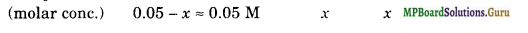Ka = $$\frac{\left[\mathrm{C}_{6} \mathrm{H}_{5} \mathrm{O}^{-}\right]\left[\mathrm{H}_{3} \mathrm{O}^{-}\right]}{\left[\mathrm{C}_{6} \mathrm{H}_{5} \mathrm{OH}\right]}$$
= $$\frac{\left[\mathrm{H}_{3} \mathrm{O}^{+}\right]^{2}}{\left[\mathrm{C}_{6} \mathrm{H}_{5} \mathrm{OH}\right]}$$
[H3O+] = $$\sqrt{\mathrm{K}_{\mathrm{a}} \times\left[\mathrm{C}_{6} \mathrm{H}_{5} \mathrm{OH}\right]}$$
= $$\sqrt{1 \times 10^{-10} \times 0.05}$$
= $$\sqrt{5 \times 10^{-12}}$$
= 2.2 × 10-6 M
[Phenate] = [H3O+] =2.2 × 10-6 M
Let α be the degree of ionization of phenol in the presence of 0.01 M sodium phenate.
C6 H5ONa → C6H5O + Na+ 0.01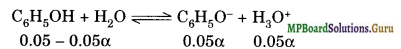[C6H5OH] = 0.05 – 0.05α
[C6H5O] = 0.01 + 0.05α = 0.01 M
[H3O+] = 0.05α
Ka = $$\frac{\left[\mathrm{C}_{6} \mathrm{H}_{5} \mathrm{O}^{-}\right]\left[\mathrm{H}_{3} \mathrm{O}^{+}\right]}{\left[\mathrm{C}_{6} \mathrm{H}_{5} \mathrm{OH}\right]}$$
= $$\frac{(0.01)(0.05 \alpha)}{(0.05)}$$
1 × 10-10 = 0.01 α
α = 1 × 10-8.

Question 45.
The first ionization constant of H2 S is 9.1 × 10-8. Calculate the concentration of HS- ion in its 0.1 M solution. How will this concentration be affected if the solution is 0.1 M in HCl also? If the second dissociation constant of H2S is 1.2 × 10-13, calculate the concentration of S under both conditions.
Solution.
Calculation of concentration of HS ions.
Case I: Let the cone. of HS be x M
H2 S ⇌ H+ + HS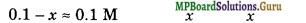Ka1 = $$\frac{\left[\mathrm{H}^{+}\right]\left[\mathrm{HS}^{-}\right]}{\left[\mathrm{H}_{2} \mathrm{~S}\right]}$$
9.1 × 10-8 = $$\frac{(x)(x)}{0.1}$$
x2 = 9.1 × 10-9
x = 9.54 × 10-5 M.
[HS] = 9.54 × 10-5 M
Case II: In the presence of 0.1 M HCl, let the concentration of HS be y M
H2 S ⇌ H+ + HS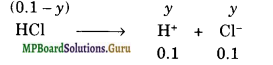[H2] =(0.1 -y)M = 0.1 M
[HS] = y
Ka1 = $$\frac{\left[\mathrm{H}^{+}\right]\left[\mathrm{HS}^{-}\right]}{\left[\mathrm{H}_{2} \mathrm{~S}\right]}$$ = $$\frac{(0.1) y}{0.1}$$
= 9.1 × 10-8 = y
[HS] = 9.1 × 10-8
Calculation of concentration of S2- ions
HS ⇌H+ + S2- Ka2 = 1.2 × 10-13

Case I: [HS] = 9.54 × 10-5 M (From first stage ionization)
[S2-] = x’ (say)
[H+] = 9.54 × 10-5 M (From first stage ionization)
Ka2 = $$\frac{\left[\mathrm{H}^{+}\right]\left[\mathbf{S}^{2-}\right]}{\left[\mathrm{HS}^{-}\right]}$$
= $$\frac{\left(9.54 \times 10^{-5}\right)\left(x^{\prime}\right)}{9.54 \times 10^{-5}}$$
1.2 × 10-13 = x’
[S2-] = 1.2 × 10-13 M.

Case II: [HS] = 9.1 × 10-8 M (From first stage ionization)
[H+] = 0.1 M (Mainly from HCl)
[S2-]=y (say)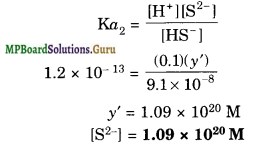Question 46.
The ionization constant of acetic acid is 1.74 × 10-5. Calculate the degree of dissociation of acetic acid in its 0.05 M solution. Calculate the concentration of acetate ion in the solution and its pH.
Solution.
CH3COOH ⇌ CH3COO + H+
Ka = $$\frac{\left[\mathrm{CH}_{3} \mathrm{COO}^{-1}\right]\left[\mathrm{H}^{+}\right]}{\left[\mathrm{CH}_{3} \mathrm{COOH}\right]}$$ = $$\frac{\left[\mathrm{H}^{+}\right]^{2}}{\left[\mathrm{CH}_{3} \mathrm{COOH}\right]}$$
It may be noted that the equilibrium concentration of the CH3COOH may be taken as the same as the initial concentration because acetic acid, being a weak acid undergoes only slight dissociation.
or [H+] = $$\sqrt{\mathrm{K}_{a}\left[\mathrm{CH}_{3} \mathrm{COOH}\right]}$$ = $$\sqrt{\left(174 \times 10^{-5}\right)\left(5 \times 10^{-2}\right)}$$
= 9.33 × 10-4M
[CH3COO] = [H+] = 9.33 × 10-4 M
pH = — log ( 9.33 × 10-4)
=4 – 0.9699
= 4- 0.97 = 3.03.

Question 47.
It has been found that the pli of a 0.01 M solution of an organic acid is 4.15. Calculate the concentration of the anion, the ionization constant of the acid and its PKa.
Solution.
HA ⇌ H+ + A
pH = – log [H+] log [H+]
= – pH = -4.15 = 5.85
[H] = antilog (.85) = 7.08 x iO M
= 7.08 x 10 M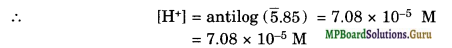[A] = [H+] = 7.08 × 10-5M
Ka = $$\frac{\left[\mathrm{H}^{+}\right]\left[\mathrm{A}^{-}\right]}{[\mathrm{HA}]}$$ = $$\frac{\left(7.08 \times 10^{-5}\right)\left(7.08 \times 10^{-5}\right)}{10^{-2}}$$
= 5.01 × 10-7
pKa = – log ka = – log (5.01 × 10-7)
= 7 – 0.699 = 6.301.Question 48.
Assuming complete dissociation, calculate the pH of the following solutions:
(a) 0.003 M HCl
(b) 0.005 M NaOH ‘
(c) 0.002 M HBr
(d) 0.002 M KOH.
Solution.
(a) HCl + aq → H+ + Cl,
∴ [H+] = [HCl] = 3 × 10-3 M,
pH = – log (3 × 10-3) = 2.52

(b) NaOH + aq → Na+ + OH
∴ [OH] = 5 × 10-3M,
[H+] = 10-14/(5 × 10-3) = 2 × 10-12 M .
pH = -log (2 × 10-12) = 11.70

(c) HBr + aq → H+ + Br
∴ [H+] = 2 × 10-3 M,
pH = – log (2 × 10-12) = 2.70

(d) KOH + aq → K+ + OH
∴ [OH] = 2 × 10-3 M,
[H+] = $$\frac{10^{-14}}{\left(2 \times 10^{-3}\right)}$$
= 5 × 10-12
pH = – log (5 × 10-12)
= 11.30.

Question 49.
Calculate the pH of the following solutions:
(a) 2 g of TIOH dissolved in water to give 2 litre of the solution.
(b) 0.3 g of Ca (OH)2 dissolved in water to give 500 mL of the solution.
(c) 0.3 g of NaOH dissolved in water to give 200 mL of the solution.
(d) 1 mL of 13.6 M HCl is diluted with water to give 1 litre of the solution.
Solution.
(a) Molar cone, of TIOH concentration
= $$\frac{2 g}{221 g \mathrm{~mol}^{-1}} \times \frac{1}{2 \mathrm{~L}}$$ = 4.52 × 10-3 M
[OH] = [TlOH] = 4.52 × 10-3 M
[H+] = $$\frac{10^{-14}}{\left(4.52 \times 10^{-3}\right)}$$
= 2.21 × 10-12 M
pH = – log (2.21 × 10-12)
= 12 – (0.3424)
= 11.66

(b) [Ca(OH)2] = $$\frac{0.3 g}{74 g \mathrm{~mol}^{-1}} \times \frac{1}{0.5 \mathrm{~L}}$$ = 8.11 × 10-3 M
Ca(OH)2 → Ca2++ 2OH
∴ [OH] = 2[Ca(OH)2) = 2 × (8.11 × 10-3) M
= 1.622 × 10-2 M
pOH = -log (1.622 × 10-2)
= 2-0.2101
= 1.79
pH = 14 – pOH
= 14-1.79 = 12.21

(c) [NaOH] = $$\frac{0.3 g}{40 g \mathrm{~mol}^{-1}} \times \frac{1}{0.2 \mathrm{~L}}$$ = 3.75 × 10-2
[OH] = 3.75 × 10-2
pOH = – log (3.75 × 10-2) = 2 – 0.0574 = 1.43
∴ pH = 14 – pOH
= 14 – 1.43 = 12.57

(d) M1V1 = M2V2
∴ 13.6 M × 1 mL = M2 × 1000 mL
M2 = 1.36 × 10-2 M
[H+] = [HCl] = 1.36 × 10-2 M, pH
= -log (1.36 × 10-2)
= 2-0.1335 = 1.87.

Question 50.
The degree of ionization of a 0.1 M bromoacetic acid solution is 0.132. Calculate the pH of the solution and the pKa of bromoacetic acid.
Solution.
BrCH2COOH ⇌ BrCH2COO + H+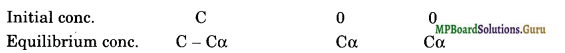Ka = $$\frac{\left[\mathrm{BrCH}_{2} \mathrm{COO}^{-}\right]\left[\mathrm{H}^{+}\right]}{\left[\mathrm{BrCH}_{2} \mathrm{COOH}\right]}$$
= $$\frac{\mathrm{C} \alpha \cdot \mathrm{C} \alpha}{\mathrm{C}(1-\alpha)}$$ = $$\frac{\mathrm{C} \alpha^{2}}{1-\alpha}$$
= Cα2 = 0.1 × (0.132)2
= 1.74 × 10-3 ;
pKa = – log Ka
= -log (1.74 × 10-3)
= 3 – 0.2405
= 2.76
[H+] = Cα= 0.1 × 0.132
= 1.32 × 10-2 M pH = – log (1.32 × 10-2)
= 2-0.1206
= 1.88.

Question 51.
The pH of 0.005 M codeine (C18 H21 NO3) solution is 9.95. Calculate the ionization constant and pKb.
Solution.
Codeine is a base. Its ionization may be represented as
B + H2O ⇌ BH+ + OH
pH = 9.95
∴pOH = 14 – 9.95
= 4.05, i.e., – log [OH] = 4.05
or log [OH] = – 4.05 = 5.95 or [OH-]
= 8.91 × 10-5
Kb = $$\frac{\left[\mathrm{BH}^{+}\right]\left[\mathrm{OH}^{-}\right]}{[\mathrm{B}]}$$
= $$\frac{\left[\mathrm{OH}^{-}\right]^{2}}{[\mathrm{~B}]}$$
= $$\frac{\left(8.91 \times 10^{-5}\right)^{2}}{5 \times 10^{-3}}$$
= 1.588 × 10-6
pKb=-log (1.588 × 10-6)
= 6-0.1987 = 5.8.

Question 52.
What is the pH of 0.001 M aniline solution? The ionization constant of aniline is 4.27 × 10-10. Calculate the degree of ionization of aniline in the solution. Also calculate the ionization constant of the conjugate acid of aniline.
Solution.
C6H5NH2 + H2O ⇌ C6H5NH3+ + OH
ka = $$\frac{\left[\mathrm{C}_{6} \mathrm{H}_{5} \mathrm{NH}_{3}{ }^{+}\right]\left[\mathrm{OH}^{-}\right]}{\left[\mathrm{C}_{6} \mathrm{H}_{5} \mathrm{NH}_{2}\right]}$$
= $$\frac{\left[\mathrm{OH}^{-}\right]^{2}}{\left[\mathrm{C}_{6} \mathrm{H}_{5} \mathrm{NH}_{2}\right]}$$
[OH] = $$\sqrt{\mathrm{K}_{\mathrm{a}}\left[\mathrm{C}_{6} \mathrm{H}_{5} \mathrm{NH}_{2}\right]}$$
= $$\sqrt{\left(4.27 \times 10^{-10}\right)\left(10^{-3}\right)}$$
= 6.534 × 10-7 M
pOH = – log [OH]
= – log (6.534 × 10-7) = 7-0.8152 = 6.18
∴ pH = 14 – 6.18 = 7.82

a = $$\sqrt{\frac{\mathrm{K}_{b}}{\mathrm{C}}}$$
= $$\sqrt{4.27 \times 10^{-10} / 10^{-3}}$$
= 6.53 × 10-4.
Ka (conjugate acid) = $$\frac{\mathrm{K}_{w}}{\mathrm{~K}_{b}}$$
= $$\frac{1 \times 10^{-14}}{4.27 \times 10^{-10}}$$
= 2.34 × 10-5 .

Question 53.
Calculate the degree of ionization of 0.05 M acetic acid if its pKa value is 4.74. How is the degree of dissociation affected when its solution also contains (a) 0.01 M (b) 0.1 M HCl?
Solution.
pKa = 4.74,
– log Ka = 4.74
or log Ka = – 4.74 = 5.26
∴ K = 1.82 × 10-5

α = $$\sqrt{\frac{\mathrm{K}_{a}}{\mathrm{C}}}$$
= $$\sqrt{\frac{\left(182 \times 10^{-5}\right)}{\left(5 \times 10^{-2}\right)}}$$
= 1.9 × 10-2
(a) In presence of HCl, due to high concentration of H+ ion, dissociation equilibrium will shift in backward direction,
In presence of 0.01 M HCl, if x is the amount dissociated, then
HCl → H+ + Cl 0.01 M
CH3 COOH ⇌ CH3COO + H+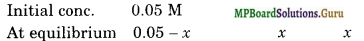[CH3COOH] = (0.05 – x) M = 0.05 M
[H+] = (0.01 +x) = 0.01 M
[CH3COO] = x M
∴ Ka = $$\frac{\left[\mathrm{CH}_{3} \mathrm{COO}^{-}\right]\left[\mathrm{H}^{+}\right]}{\left[\mathrm{CH}_{3} \mathrm{COOH}\right]}$$
= $$\frac{x(0.01)}{0.05}$$
or $$\frac{x}{0.05}$$ = $$\frac{\mathrm{K}_{a}}{0.01}$$
= $$\frac{1.82 \times 10^{-5}}{10^{-2}}$$
= 1.82 × 10-3
∴ α = 1.82 × 10-3
(b) In the presence of 0.1 M HCl, Ify is the amount of acetic acid dissociated, then at equilibrium
[CH3COOH] = 0.05 -y – 0.05 M
[CH3COOH] = y,
[H+] = 0.1 M + y = 0.1 M
Ka = $$\frac{\left[\mathrm{CH}_{3} \mathrm{COO}^{-}\right]\left[\mathrm{H}^{+}\right]}{\left[\mathrm{CH}_{3} \mathrm{COOH}\right]}$$
= $$\frac{y(0.1)}{0.05}$$
or $$\frac{y}{0.05}$$ = $$\frac{\mathrm{K}_{a}}{0.1}$$ = $$\frac{182 \times 10^{-5}}{10^{-1}}$$
= 1.82 × 10-4
∴ α = 1.82 × 10-4

Question 54.
The ionization constant of dimethylamine is 5.4 × 10-4. Calculate its degree of ionization in its 0.02 M solution. What percentage of dimethylamine is ionized if the solution is also 0.1 M NaOH?
Solution.
α = $$\sqrt{\frac{\mathrm{K}_{b}}{\mathrm{C}}}$$
= $$\sqrt{\frac{\left(5.4 \times 10^{-4}\right)}{\left(2 \times 10^{-2}\right)}}$$
= 0.164
In presence of 0.1 M NaOH, ifx is the amount of dimethyl amine dissociated, then
(CH3)2NH + H2O ⇌ (CH3)2NH2 + OH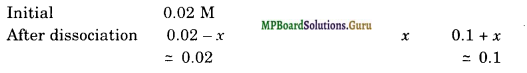[(CH3)2 NH] = (0.02 -x) M = 0.02 M ‘
[(CH3)2 NH2+] = X M
[OH] = [0.1 + x]M = 0.1 M

Kb = $$\frac{\left[\left(\mathrm{CH}_{3}\right)_{2} \mathrm{NH}_{2}{ }^{+}\right]\left[\mathrm{OH}^{-}\right]}{\left[\left(\mathrm{CH}_{3}\right)_{2} \mathrm{NH}\right]}$$
= $$\frac{x(0.1)}{0.02}$$
or $$\frac{x}{0.02}$$ = $$\frac{\mathrm{K}_{b}}{0.1}$$
= $$\frac{5.4 \times 10^{-4}}{10^{-1}}$$
= 5.4 × 10-3
∴ α = 5.4 × 10-3
Percent dissociation = α × 100
= 5.4 × 10-3 × 100
= 0.54.

Question 55.
Calculate the hydrogen ion concentration in the following biological fluids whose pH are given below:
(a) Human muscle-fluid 6.83
(b) Human stomach fluid 1.2
(c) Human blood 7.38
(d) Human saliva 6.4.
Solution.
(a) – log [H+] = 6.83
log [H+] = -6.83 = 7.17
[H+] = antilog (7.17)
= 1.48 × 10-7 M

(b) -log [H+] = 1.2
log [H+] = -1.2 = 2.8
[H+] = antilog (2.8)
= 6.31 × 10-2 M

(c) -log [H+] = 7.38
log [H+] = -7.38 = 8.62
[H+] = antilog (8.62)
= 4.17 × 10-8 M

(d) -log [H+] = 6.4
log [H+] = -6.4 = 7.6
[H+] = antilog (7.6)
= 3.98 × 10-7 M.Question 56.
The pH of (i) milk, (ii) black coffee, (iii) tomato juice, (iv) lemon juice and (v) egg white are 6.8, 5.0, 4.2, 2.2 and 7.8 respectively. Calculate corresponding hydrogen ion concentration in each.
Solution:
(i) pH of milk = 6.8
-log[H+] = 6.8 or log [H+] = 6.8 = 7.2
[H+] = antilog (7.2) = 1.585 × 10-7

(ii) pH of black coffee = 5.0
-log[H+] = 5.0 or log [H+] = -5.00 = 5.00
[H+] = antilog (5.00) = 1 × 10-5 M

(iii) pH of tomato juice = 4.2
-log[H+] = 4.2 or log [H+] = -4.2 = 5.8
[H+] = antilog (5.8) = 6.31 × 10-5 M
similarly , [H+] in (iv) lemon jucie is 6.31 ×10-3 M and in (v) egg white is 1.585 × 10-8 M.

Question 57.
If 0.56 g of KOH is dissolved in water to give 200 mL of solution at 298 K, calculate the concentrations of potassium, hydrogen and hydroxyl ions. What is its pH?
Solution.
Amount of KOH in 200 mL of solution = 0.56 g
Amount of KOH per liter of solution
= $$\frac{0.56}{200}$$ × 1000 = 2.8 g
Molar mass of KOH = 56g mol-1
[KOH] = $$\frac{28}{56}$$ mol L-1
= 0.05 mol L-1 or 0.05 M

Since KOH is a strong base, it is completely ionized in aqueous solution.
∴ [K+] = [KOH] = 0.05 M
[OH] = [KOH] = 0.05 M
[H+]= $$\frac{\mathrm{K}_{w}}{\left[\mathrm{OH}^{-}\right]}$$
= $$\frac{1 \times 10^{-14}}{0.05}$$ = 2.0 × 10-13 M
pH = -log [H+]
= – log (2.0 × 10-13)
= 13 – log 2
= 12.7 .

Question 58.
The solubility of Sr(OH)2 at 298 K is 19.23 g/L of solution. Calculate the concentrations of strontium and hydroxyl ions and the pH of the solution.
Solution.
Molar mass of Sr(OH)2
= 87.6 + 16×2 +1×2 = 121.6 g mol L-1
Solubility of Sr(OH)2 = 19.23 g/L
= $$\frac{19.23 \mathrm{~g} / \mathrm{L}}{121.6 \mathrm{~g} / \mathrm{mol}}$$ = 0.158 molL-1
[Sr2+] = [Sr(OH)2] = 0.158 mol L-1
[OH] = 2[Sr(OH)2] = 2 × 0.158 mol L-1
= 0.316 mol L-1
[H+] = $$\frac{\mathrm{K}_{w}}{\left[\mathrm{OH}^{-}\right]}$$ = $$\frac{1 \times 10^{-14} \mathrm{~mol}^{2} \mathrm{~L}^{-2}}{0.316 \mathrm{~mol} \mathrm{~L}^{-1}}$$
= 3.165 × 10-14 mol L-1
pH = – log [H+]
= -log (3.165 ×10-14)
= 14 – log 3.165 = 13.5.

Question 59.
The ionization constant of propanoic acid is 1.32 × 10-5. Calculate the degree of ionization of the acid in its 0.05 M solution and also its pH. What will be its degree of ionization if the solution is 0.01 M in HCl also?
Solution.
Let us represent propanoic acid as HA. Let its degree of ionization be a.
HA + H2O ⇌ H3O+ + A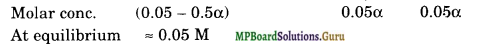Ka = $$\frac{\left[\mathrm{H}_{3} \mathrm{O}^{+}\right]\left[\mathrm{A}^{-}\right]}{[\mathrm{HA}]}$$ = $$\frac{(0.05 \alpha)(0.05 \alpha)}{(0.05)}$$ = 0.05 α 2
α = $$\sqrt{\frac{\mathrm{Ka}}{0.05}}$$
= $$\sqrt{\frac{1.32 \times 10^{-5}}{0.05}}$$
= 1.63 × 10-2
[H3O+] = 0.05 α
= 0.05 × 1.63 × 10-2
= 8.15 × 10-4 M
pH = – log [H3O+]
= – log 8.15 × 10-4
= 4-log 8.15
= 4-0.91
= 3.09
In the presence of 0.01 M HCl, let a’ be the degree of ionization
[H3O+] = 0.01 M
[A] = 0.05 α’ M
[HA] = 0.05 – 0.05 α’ = 0.05 M
Kα = $$\frac{\left[\mathrm{H}_{3} \mathrm{O}^{+}\right]\left[\mathrm{A}^{-}\right]}{[\mathrm{HA}]}$$
= $$\frac{(0.01)\left(0.05 \alpha^{\prime}\right)}{(0.05)}$$
α’ = $$\frac{\mathrm{K} a}{0.01}$$
= $$\frac{1.32 \times 10^{-5}}{0.01}$$
= 1.32 × 10-3.

Question 60.
The pH of 0.1 M solution of cyanic acid (HCNO) is 2.34. Calculate the ionization constant of the acid and its degree of ionization in the solution.
Solution.
– log [H3O+] = 2.34
or log [H3O+] = – 2.34 = 3.66
[H3O+] = antilog (3.66)
= 4.57 × 10-3 M
[H3O+] = Cα
∴ α = $$\frac{\left[\mathrm{H}_{3} \mathrm{O}^{+}\right]}{\mathrm{C}}$$
= $$\frac{4.57 \times 10^{-3} \mathrm{M}}{0.1 \mathrm{M}}$$
= 4.57 × 10-2
[H3O+] = $$\sqrt{\mathrm{K}_{a} \times \mathrm{C}}$$
∴ Ka = $$\frac{\left[\mathrm{H}_{3} \mathrm{O}^{+}\right]^{2}}{\mathrm{C}}$$
= $$\frac{\left(4.57 \times 10^{-3}\right)^{2}}{0.1}$$
= 2.09 × 10-4.

Question 61.
The ionization constant of nitrous acid is 4.5 × 10-4. Calculate the pH of 0.04 M sodium nitrite solution and also its degree of hydrolysis.
Solution.
Sodium nitrite is a salt of strong base and weak acid. Its degree of hydrolysis, h is given by the relation:
h = $$\sqrt{\frac{\mathrm{K}_{u^{-}}}{\mathrm{K}_{a} \times \mathrm{C}}}$$
=$$\sqrt{\frac{1 \times 10^{-14}}{4.5 \times 10^{-4} \times 0.04}}$$
= $$\sqrt{5.56 \times 10^{-10}}$$
= 2.36 × 10-5
pH = $$\frac{1}{2}\left[\mathrm{pK}_{w}+\mathrm{pK}_{a}+\log \mathrm{C}\right]$$
= $$\frac{1}{2}\left[14-\log \left(4.5 \times 10^{-4}\right)+\log (0.04)\right]$$
= $$\frac{1}{2}[14+3.3468-1.398]$$
= 7.97

Question 62.
A 0.02 M solution of pyridinium hydrochloride has pH = 3.44. Calculate the ionization constant of pyridine.
Solution.
Pyridinium hydrochloride is a salt of a weak base [pyridine and a strong acid (HCl)]. The pH of an aqueous solution of this salt is given by the relation:
PH = $$\frac{1}{2}\left[p \mathrm{~K}_{w}-p \mathrm{~K}_{b}-\log \mathrm{C}\right]$$
3.44 = $$\frac{1}{2}\left[14+\log K_{b}-\log (0.02)\right]$$
6.88 = 14 + log Kb + 1.7
log Kb = – 882 = 9.18
Kb = 1.5 × 10-9.

Question 63.
Predict if the solutions of the following salts are neutral, acidic or basic: NaCl, KBr, NaCN, NH4NO3, NaNO2 and KF.
Solution.
Neutral solutions: NaCl, KBr
Acidic solutions: NH4NO3
solutions Basic solutions:NaCN, NaNO2 and KF.

Question 64.
The ionization constant of chloroacetic acid is 1.35 × 10-3. What will be the pH of 0.1 M acid and its 0.1 M sodium salt solution?
Solution.
(i) Calculation of pH of 0.1 M acid.
[H+] = $$\sqrt{\mathrm{K}_{a} \times \mathrm{C}}$$
= $$\sqrt{135 \times 10^{-3} \times 0.1}$$
= 1.16 × 10-2 M.
pH = – log [H+] = – log (1.16 × 10-2) = 2-log 1.16 = 2-0.06
= 1.94.

(ii) Calculation of pH of 0.1 M sodium salt of the acid
This would be a salt of strong base and weak acid. Its pH would be given by the relation:
pH = $$\frac{1}{2}\left[\mathrm{pK}_{w}+\mathrm{pK}_{a}+\log \mathrm{C}\right]$$
= $$\frac{1}{2}\left[14-\log \left(1.35 \times 10^{-3}\right)+\log (0.1)\right]$$
= $$\frac{1}{2}[14+(3-\log 1.35)-1]$$
= $$\frac{1}{2}[14+(3-0.1303)-1]$$
= 7.94.

Question 65.
Ionic product of water at 310 K is 2.7 × 10-14. What is the pH of neutral water at this temperature?
Solution.
Kw= [H3O+] [OH]
For pure water,
[H3O+] = [OH]
Kw = [H3O+]2
[H3O+] =$$\sqrt{\mathrm{K}_{w}}$$ = $$\sqrt{2.7 \times 10^{-14}}$$
= 1.643 × 10-7 mol L-1
pH = – log [H3O+]
= – log (1.643 × 10-7) = 7 – log 1.643
= 7-0.2156
= 6.78.Question 66.
Calculate the pH of the resultant mixtures:
(а) 10 mL of 0.2 M Ca(OH)2 + 25 mL of 0.1 M HCl
(б) 10 mL of 0.01 M H2SO4 + 10 mL of 0.01 M Ca (OH)2
(c) 10 mL of 0.1 M H2SO4 + 10 mL of 0.1 M KOH.
Solution.
(a) 10 mL of 0.2 M Ca(OH)2 = 10 × 0.2 millimoles = 2 millimoles of Ca(OH)2 = 25 mL of 0.1 M HCl = 25 × 0.1 millimoles = 2.5 millimoles of HCl
Ca(OH)2 + 2 HCl → CaCl2 + 2 H2O
1 millimole of Ca(OH)2 reacts with 2 millimoles of HCl
∴ 2.5 millimoles of HCl will react with 1.25 millimoles of Ca(OH)2
∴ Ca(OH)2 left unreacted = 2 – 1.25 = 0.75 millimoles

Total volume of the solution = (10 + 25) mL = 35 mL
∴ Molarity of Ca(OH)2 in the mixture solution
= $$\frac{0.75}{35}$$ M = 0.0214 M
∴ [OH-] = 2 × 0.0214 M
= 0.0428 M = 4.28 × 10-2 M .
pOH = – log (4.28 × 10-2)
= 2 – 0.6314
= 1.3686
= 1.37
∴ pH = pKw – pOH
= 14-1.37
= 12.63

(b) 10 mL of 0.01 MH2SO4
= 10 × 0.01 millimole
= 0.1 millimole
10 mL of 0.01 M Ca(OH)2 = 10 × 0.01 millimole
= 0.1 millimole
Ca(OH)2 + H2SO4 → CaSO4 + 2H2O
∴ 1 mole of Ca(OH)2 reacts with 1 mole of H2SO4
∴ 0.1 millimole of Ca(OH)2 will react completely with 0.1 millimole of H2SO4.
Hence, solution will be neutral with pH = 7.0

(c) 10 mL of 0.1 M H2SO4 = 1 millimole
10 mL of 0.1 M KOH = 1 millimole
2 KOH + H2SO4 → K2SO4 + 2 H2O
1 millimole of KOH will react with 0.5 millimole of H2SO4
∴ H2SO4 left unreated = 1 – 0.5 = 0.5 millimole
Volume of reaction mixture = 10 + 10 = 20 mL
∴ Molarity of H2SO4 in the mixture solution
=$$\frac{0.5}{20}$$ =2.5 × 10-2 M
[H+] = 2 × 2.5 × 10-2
= 5 × 10-2M
pH = – log (5 × 10-2)
= 2 – 0.699
= 1.3

Question 67.
Determine the solubilities of silver chromate, barium chromate, ferric hydroxide, lead chloride and mercurous iodide at 298 K. Given Ksp values: Ag2CrO4 = 1.1 × 10-12,
BaCrO4 = 1.2 × 10-10, Fe(OH)3 = 1.0 × 10-38
PbCl2 = 1.6 × 10-5
Hg2I2 = 4.5 × 10-29
Determine also the molarities of individual ions.
Solution.
Ag2Cr04(s) ⇌ 2Ag+(aqr) + CrO42-(ag);
Ksp = [Ag+]2[CrO>sub>42-] = (2s)2(s) = 4s3
∴ s3 = $$\frac{\mathrm{K}_{s p}}{4}$$
s3 = $$\frac{\left(1.1 \times 10^{-12}\right)}{4}$$
= 0.275 × 10-12
= 2.75 ×10-13
∴ s = 6.5 × 10-5 mol L-1
[Ag+] = 2 10-5 mol L-1
[Ag+] = 2 6.5 × 10-5 M
= 13.0 × 10-5 M
= 1.30 × 10-4 M
[CrO42-] = 6.5 × 10-5 M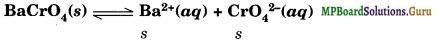Ksp = [Ba2+] [CrO42- = (s) (s) = s2
s = $$\sqrt{\mathrm{K}_{s p}}$$
= $$\sqrt{1.2 \times 10^{-10}}$$
= 1.1 × 10-5 M
[Ba2+] = 1.1 × 10-5 M, [CrO42-] = 1.1 × 10-5 M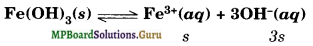Ksp = [Fe3+] [OH]3 = (s) (3s)3 = 27s4
s4 = $$\frac{\mathrm{Ksp}}{27}$$ = $$\frac{1.0 \times 10^{-38}}{27}$$
s = 1.39 × 10-10
[Fe3+] = 1.39 × 10-10 M, [OH]
= 3 × 1.39 ×10-10 M = 4.17 × 10-10 M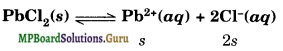Ksp = [Pb2+][Cl]2 = (s) (2s)2 = 4s3
4s3 = Ksp = 1.6 × 10-5
s3 = 4 × 10-6 or s = 1.59 × 10-2 M
[Pb2+] = 1.59 × 10-2 M
[Cl] = 2 × 1.59 × 10-2 M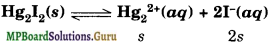= 2 × 2.24 × 10-10 M
= 4.48 × 10-10 M

Question 68.
The solubility product constant of Ag2CrO4 and AgBr are 1.1 × 10-12 and 5.0 × 10-13 respectively. Calculate the ratio of the molarities of their saturated solutions.
Solution.
Let x be the solubility of Ag2CrO4
Ag2CrO4 (s) ⇌ 2Ag+(ag) + CrO42-(ag)
2x x
Ksp = [Ag+]2[CrO42-]
= (2x)2(x) = 4x3
x3 = $$\frac{K s p}{4}$$ = $$\frac{11 \times 10^{-12}}{4}$$
x = 6.5 × 10 -5M

Let y be the solubility of AgBr
AgBr ⇌ Ag+(aq) + Br(aq)
Ksp = [Ag+] [Br] = y × y = y2
5.0 × 10-13 = y2 or y = 7.07 × 10-7 M
The ratio of molarit les of their saturated solutions
= $$\frac{6.5 \times 10^{5} \mathrm{M}}{7.07 \times 10^{-7} \mathrm{M}}$$
= 91.9.
Thus, silver chromate is 91.9 times more soluble than silver bromide.

Question 69.
Equal volumes of 0.002 M solutions of sodium iodate and copper chlorate are mixed together. Will it lead to precipitation of copper iodate? For copper iodate K = 7.4 × 10-7.
Solution.
When equal volumes of sodium iodate and copper chlorate are mixed, the molar concentrations of both the solutes would be reduced to half i.e., 0.001 M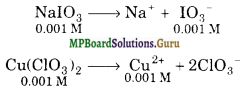∴ After mixing, [IO3] = [NaIO3] = 0.001 M
[Cu2+] = [Cu(IO3)2] = 0.001 M
Solubility equilibrium for copper iodate may be written as,
[Cu(IO3)2](s) ⇌ Cu2+(ag) + 2IO3(ag)
Ionic product of copper iodate = [Cu2+][IO3]2 = (0.001) (0.001)2 = 1 × 10-9
Since ionic product (1 × 10-9) is less than Ksp (7.4 × 10-7), therefore, no precipitation will take place.

Question 70.
The ionization constant of benzoic acid is 6.46 × 10-5 and Ksp for silver-benzoate is 2.5 × 10-13. How many times is silver benzoate more soluble in a buffer of pH 3.19 compared to its solubility in pure water?
Solution.
Solubility in pure water:
Suppose solubility of silver benzoate in pure water is x mol Lr1.
C6H5COOAg ⇌ C6H5COO (ag) + Ag+(ag)
Ksp = [ C6H5COO] [Ag+] = (x) (x) = x2
x2 = K = 2.5 × 10-13
x = 5 × 10-7mol L-1

Solubility in a buffer of pH = 3.19
– log [H3O+] = pH = 3.19
log [H3O+] = -3.19 = 4.81
[H3O+] = 6.457 × 10-4 M
C6H5COOH + H2O ⇌ C6H5COO + H3O+

Ka = $$\frac{\left[\mathrm{C}_{6} \mathrm{H}_{5} \mathrm{COO}^{-}\right]\left[\mathrm{H}_{3} \mathrm{O}^{+}\right]}{\left[\mathrm{C}_{6} \mathrm{H}_{5} \mathrm{COOH}\right]}$$
$$\frac{\left[\mathrm{C}_{6} \mathrm{H}_{5} \mathrm{COOH}\right]}{\left[\mathrm{C}_{6} \mathrm{H}_{5} \mathrm{COO}^{-}\right]}$$ = $$\frac{\left[\mathrm{H}_{3} \mathrm{O}^{+}\right]}{\mathrm{K}_{a}}$$ = $$\frac{6.457 \times 10^{-4}}{6.46 \times 10^{-5}}$$ = 10
Thus, in a buffer solution having pH = 3.19, the ratio of concentrations of benzoic acid and benzoate ion would remain about 10.
Suppose solubility of silver benzoate in the buffer solution isy mol L-1, then [Ag+] = y and [C6H5COO + [C6H5COOH] = y
1 + $$\frac{\left[\mathrm{C}_{6} \mathrm{H}_{5} \mathrm{COOH}\right]}{\left[\mathrm{C}_{6} \mathrm{H}_{5} \mathrm{COO}^{-}\right]}$$ = $$\frac{y}{\left[\mathrm{C}_{6} \mathrm{H}_{5} \mathrm{COO}^{-}\right]}$$
1 + 10 = $$\frac{y}{\left[\mathrm{C}_{6} \mathrm{H}_{5} \mathrm{COO}^{-}\right]}$$
[C6H5COO] = $$\frac{y}{11}$$
KSp=[C6H5COO][Ag+] = ($$\frac{y}{11}$$ ) (y)
2.5 × 10-23 = $$\frac{y^{2}}{11}$$
y2 = 2.5 × 10-13 × 11 or y = 1.66 × 10-6
Thus, solubility of silver benzoate in a buffer solution of pH 3.19 is 1.66 × 10-6mol L-1
$$\frac{y}{x}$$ = $$\frac{166 \times 10^{-6}}{5 \times 10^{-7}}$$ = 3.32
Thus, silver benzoate is 3.32 time more soluble in the given buffer solution than in pure water.

Question 71.
What is the maximum concentration of equimolar solutions of ferrous sulphate and sodium sulphide so that when mixed in equal volumes, there is no precipitation of iron sulphide? For iron sulphide, Ksp = 6.3 × 10-18.
Solution.
Suppose the maximum concentration of each of two solutions be x mol L-1. After mixing equal volumes the concentrations of both the solutes would be reduced to x/2.
∴ After mixing,
[FeSO4] = [Na2S] = $$\frac{x}{2}$$ M
[Fe2+] = [FeSO4]= $$\frac{x}{2}$$ M
[S2-] = [Na2S] = |M

FeS(s) ⇌ [Fe2+](aq) + [S2-](aq)
Ksp = [Fe2+][S2-]
6.3 × 10-18 = $$\left(\frac{x}{2}\right)\left(\frac{x}{2}\right)$$
x2 = 4 ×6.3 × 10-18
x = 5.02 × 10-9
Thus, there would be no precipitation of iron sulphide so long as concentrations of the two solutions are equal to or less than 5.02 × 10-9 M.Question 72.
What is the minimum volume of water required to dissolve 1 g of calcium sulphate at 298 K. For calcium sulphate, Ksp = 9.1 × 10-6.
Solution.
CaSO4(s) ⇌ Ca2+(aq) + SO42-(aq)
If s is the solubility of CaSO4 in moles Lr1, then Ksp = [Ca2+] [SO42-] = s2
or s=$$\sqrt{\mathrm{K}_{s p}}$$
= $$\sqrt{9.1 \times 10^{-6}}$$
= 3.02 × 10-3 mol L-1
= 3.02 × 10-3 × 136 g L-1
= 0.411 gL-1
For dissolving 0.411 g calcium sulphate, water required = 1 L
∴ For dissolving 1 g calcium sulphate, water required
= $$\frac{1}{0.411}$$ L
= 2.43 L.

Question 73.
The concentration of sulphide ion in 0.1 M HCl solution saturated with hydrogen sulphide is 1.0 × 10-19 M. If 10 mL of this is added to 5 mL of 0.04 M solution of the following: FeSO4, MnCl2, ZnCl2 and CdCl2, in which solutions will precipitation take place?
Ksp values : FeS (6.3 × 10-18), MnS (2.5 × 10-13),
ZnS (1.6 × 10-24), CdS (8.0 × 10-27).
Solution.
For precipitation to occur the calculated ionic product must exceed the Ksp value.
Before mixing:
[S2-] = 1.0 × 10-19 M, [M2+] = 0.04 M
Volume = 10 mL, Volume = 5 mL
After mixing:
[S2-] = ?, [M2+] = ?
Volume = (10 + 5) mL = 15 mL
[S2-] = $$\frac{1.0 \times 10^{-19} \times 10}{15}$$ = 6.67 × 10-20 M
[M2+] = $$\frac{0.04 \times 5}{15}$$ = 1.33 × 10-2 M
For each of these salts, ionic product can be calculated from the following expression: Ionic product = [M2+][S2-] = (1.33 × 10-2) (6.67 × 10-20)
= 8.87 × 10-2
Since the value of ionic product is greater than the Ksp values for CdS and ZnS, therefore, precipitation will take place in CdCl2 and ZnCl2 solutions.

### MP Board Class 11 Chemistry Equilibrium Important Questions and Answers

Question 1.
Why solubility of a gas in water decreases with increase in temperature?
Dissolution of gas in water is an exothermic process.

Question 2.
What is the relationship between Kc and ΔG°?
ΔG° = -2.303 RT log Kc.

Question 3.
What is the conjugate base of CH3OH?
CH3O (Methoxide ion).

Question 4.
What is meant by autoprotolysis of water?
Self ionization of water is also known as autoprotolysis of water
H2O + H2O ⇌ H3O+ + OH

Question 5.
What is the effect of temperature on Kw? What are its units?
Kw increases with increase in temperature. Units of Kw: mol2 L-2.

Question 6.
Why ammonia is termed as a base although it does not have OH ions in it?
The basic nature of it is due to its ability to act as a donor of electron pair.

Question 7.
Why is aqueous solution of CuSO4 acidic?
Due to hydrolysis, the net hydrolytic reaction is
Cu2+ + SO42- + 2H2O ⇌ Cu(OH)2 + 2H+ + SO42-

Question 8.
Write conjugate bases of HCN, HCO3, NH3, HN3.
CN, CO32-, : NH2, N3 (azide ion)

Question 9.
Write an equation to show autoprotic nature of water.
H2O + H2O ⇌ H3O+ + OH.

Question 10.
How is degree of dissociation of a weak base related to its molarity?
If M is the molarity, then α ≈ $$\sqrt{\mathrm{K}_{a} / \mathrm{M}}$$

Question 11.
How pH, pOH and pKw are related to one another?
pKw = pH + pOH : At 25°C pKw = 14.Question 12.
What is the relation between dissociation constant of an acid and dissociation constant of its conjugate base?
Ka x Kb = Kw.

Question 13.
How does the degree of ionization of a weak acid change with increase in concentration?
Degree of ionization of a weak acid decreases with increase in concentration.

Question 14.
Write solubility product expression for BaCr04.
Ksp = [Ba2+][CrO42-]

Question 15.
If solubility of AgCl in water is x at 298 K, what is the value of Ksp for AgCl at 298 K?
Ksp = [Ag+] [Cl ] = x × x = x2.

Question 16.
What is the maximum value of ionic product of a salt at a particular temperature?
The maximum value of ionic product is equal to solubility product constant, Ksp.

Question 17.
What is meant by concentration quotient of chemical reaction? Under what conditions, is it equal to equilibrium constant?
Q is equal to K at the equilibrium point.

Question 18.
What is the meaning of notation [A]x as used in the expression of equilibrium constant?
Square bracket enclosed around A represents molar concentration of A. x represents stoichiometric co-efficient of A in the balanced equation.

Question 19.
Write expression and units of Kp for the reaction
A2(g) + 3B2 ⇌ 2AB3(g)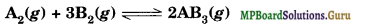Question 20.
Can equilibrium be achieved between water and its vapours in an open beaker?
No, equilibrium gets established when reversible process is carried out in a closed vessel.Question 21.
What is the effect of a catalyst on equilibrium state?
A catalyst has no effect on equilibrium state. It simply hastens the attainment of equilibrium.

Question 22.
Which is more acidic, a solution having a pH of 4 or one having pH of 3?
The solution having lower value of pH is more acidic as it contains higher concentration of hydronium ions. Hence, the solution with pH 3 is more acidic.

Question 23.
Write an equation in which CH3OH acts as a base and another in which it acts as an acid.
As Acid : CH3OH + H2O ⇌ CH3O + H3O+
As Base : CH3OH + H3O+ ⇌ CH3OH2+ + H2O.

Question 24.
Write the equation for the ionization of aniline, C6H5NH2, in aqueous solution. Write the expression for Knife.
C6H5NH2 ⇌ C6H5NH3+ + OH
Kb = $$\frac{\left[\mathrm{C}_{6} \mathrm{H}_{5} \mathrm{NH}_{3}^{+}\right]\left[\mathrm{OH}^{-}\right]}{\left[\mathrm{C}_{6} \mathrm{H}_{5} \mathrm{NH}_{2}\right]}$$

Question 25.
State the formula and the name of the conjugate base of each of the following acids:
(i) H3O+ (ii) HSO4 (iii) NH4+ (iv) HF.
(i) H2O
(ii) SO42-
(iii) NH3
(iv) F

Question 26.
State the formula and name of the conjugate acid of each of the following bases:
(i) OH
(ii) HPO42-
(iii) H2PO4
(iv) CH3NH2
(i) H2O
(ii) H2PO4
(iii) H3PO4
(iv) CH3NH3+

Question 27.
Decide whether solutions of the following salts are acidic, basic or neutral:
(i) Sodium acetate
(ii) Ammonium acetate
(iii) Ferric nitrate
(i) basic
(ii) almost neutral
(iii) acidic.Question 28.
Equilibrium constants for certain reaction at two temperatures 20°C and 50°C are respectively 1 x 10-2 and 1 x 10-3. Can it help in predicting the exothermic or endothermic nature of the forward reaction?
At high temperature, the value of K decreases indicating that the reaction occurs in the backward direction. Now according to Le-Chatelier’s principle raising the temperature shifts equilibrium in the endothermic side. Thus, reaction is endothermic in backward direction. Hence, forward reaction should be exothermic.

Question 29.
On dissolving ammonium chloride in water, cooling effect is caused. Predict on the basis of Le-Chatelier’s principle the effect of temperature on the solu¬bility of ammonium chloride.
NH4Cl(S) + water ⇌ NH4Cl(aq); ∆H = +ve. Increase in temperature pushes the equilibrium to endothermic side (forward side) i.e., the solubility of NH4Cl increases by increasing the temperature.

Question 30.
Why do we sweat more on a humid day?
On a humid day, there is a large amount of water vapour in air around us. Therefore, evaporation of water from the skin does not occur quickly. Hence, water vapours do not dry up easily causing sweating.

Question 31.
Name the factors on which equilibrium constant depends.
The value of equilibrium constant of a reaction depends on the following:

• temperature
• mode of expressing the concentrations, e.g., Kp and K, values are different.
• forms of equation which describe the equilibria

Question 32.
Why solubility of CO2 decreases with rise in temperature?
CO2(g) + Water ⇌ CO2(aq)
The process involves decrease of randomness. Hence, it must be exothermic in forward direction. Now increase of temperature pushes it in the backward side thereby decreasing the solubility.

Question 33.
Zn2+ are precipitated by passing H2S in ammoniacal solution but they are not precipitated from acidic solution. Explain.
Due to removal of H+ ions by NH3 which shifts the dissociation of H2S in forward direction
H2S ⇌ 2H+ + S2-
NH3 + H+ → NH4+
In acidic solution dissociation of H2S is suppressed due to common ion effect
H2S ⇌ 2H+ + S2-
HCl → H+ Cl
Due to low cone, of S2” ions Qsp does not exceed Kgp for ZnS and it is not precipitated.

Question 34.
What is the difference between steady-state and equilibrium state as applied to chemical systems?